Question

Signals And Systems

5. Using the feedback analysis method, answer the following questions for each circuit:

a.Classify the feedback topology and the type of amplifier.

b. Draw the small-signal equivalent circuit used for calculating the open-loop gain,input resistance, and output resistance, i.e., break the feedback path and include the loading effects of the feedback network.

c. Write expressions for the circuit's reverse transmission factor, B, and open-loop gain, A.

d. Assuming that the circuit has sufficient loop gain, write the approximate expression for the amplifier's closed loop gain, As, and evaluate it.

e. Based on your answer to part d, approximate the feedback amplifier's voltage gain.Verified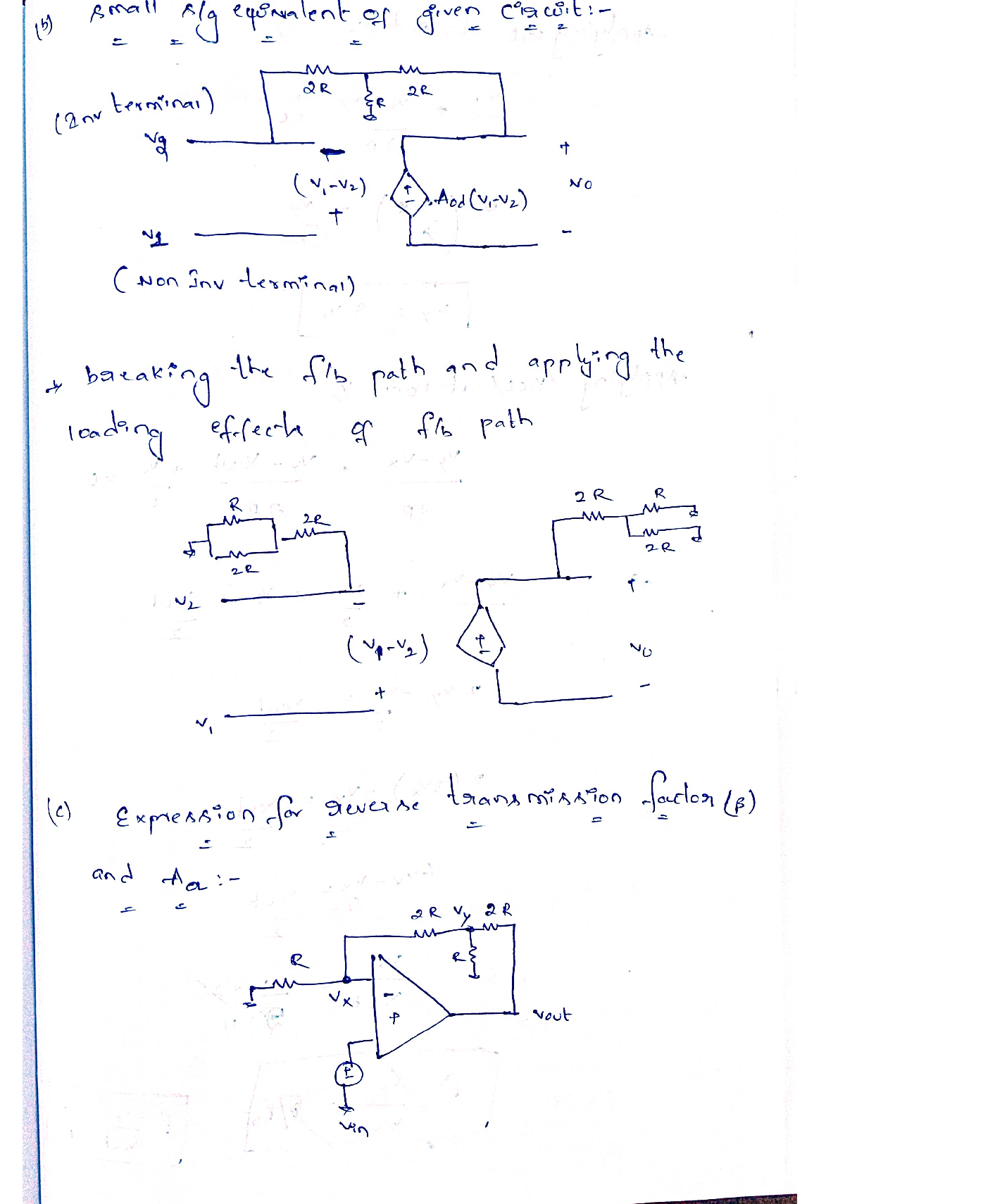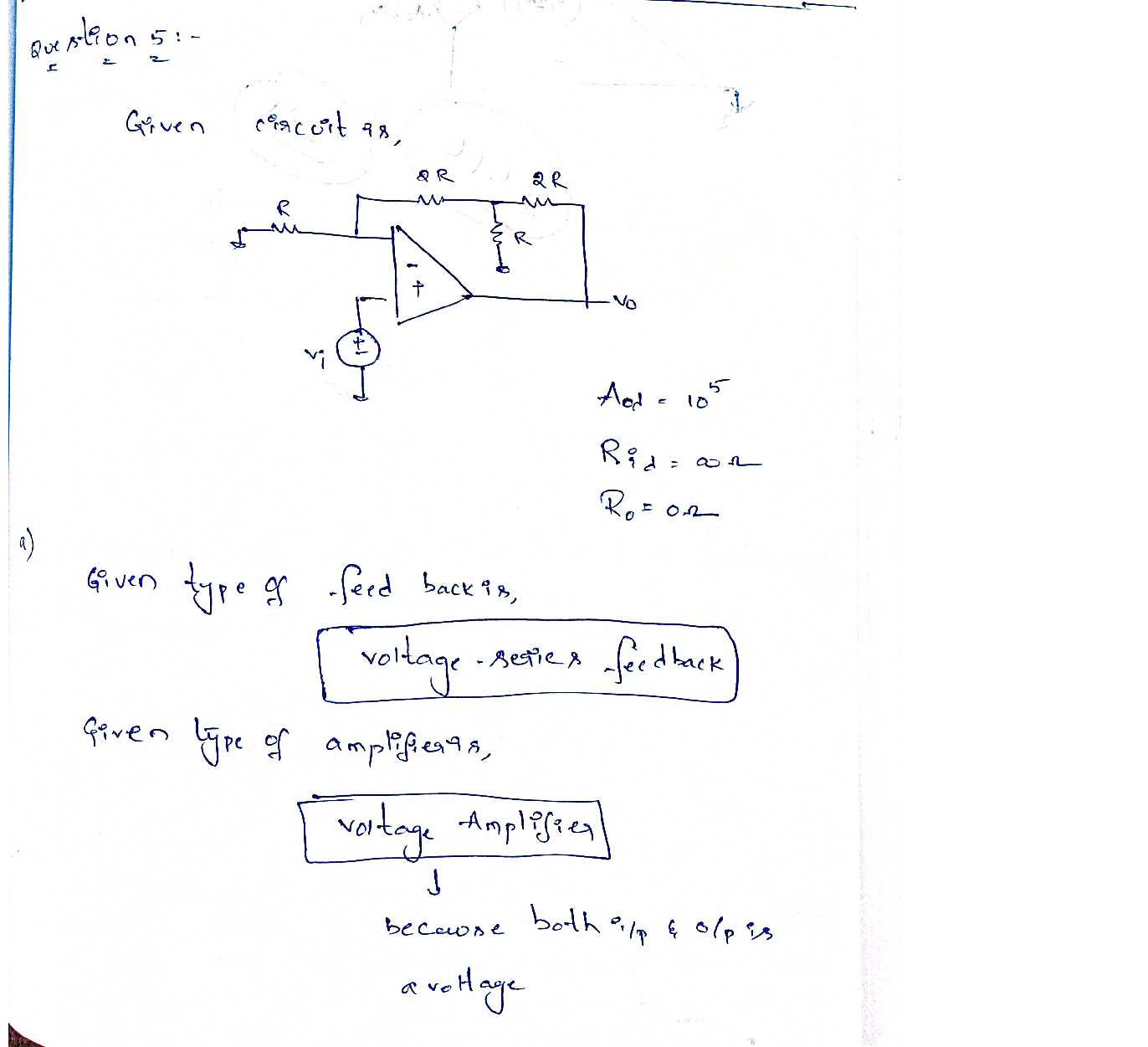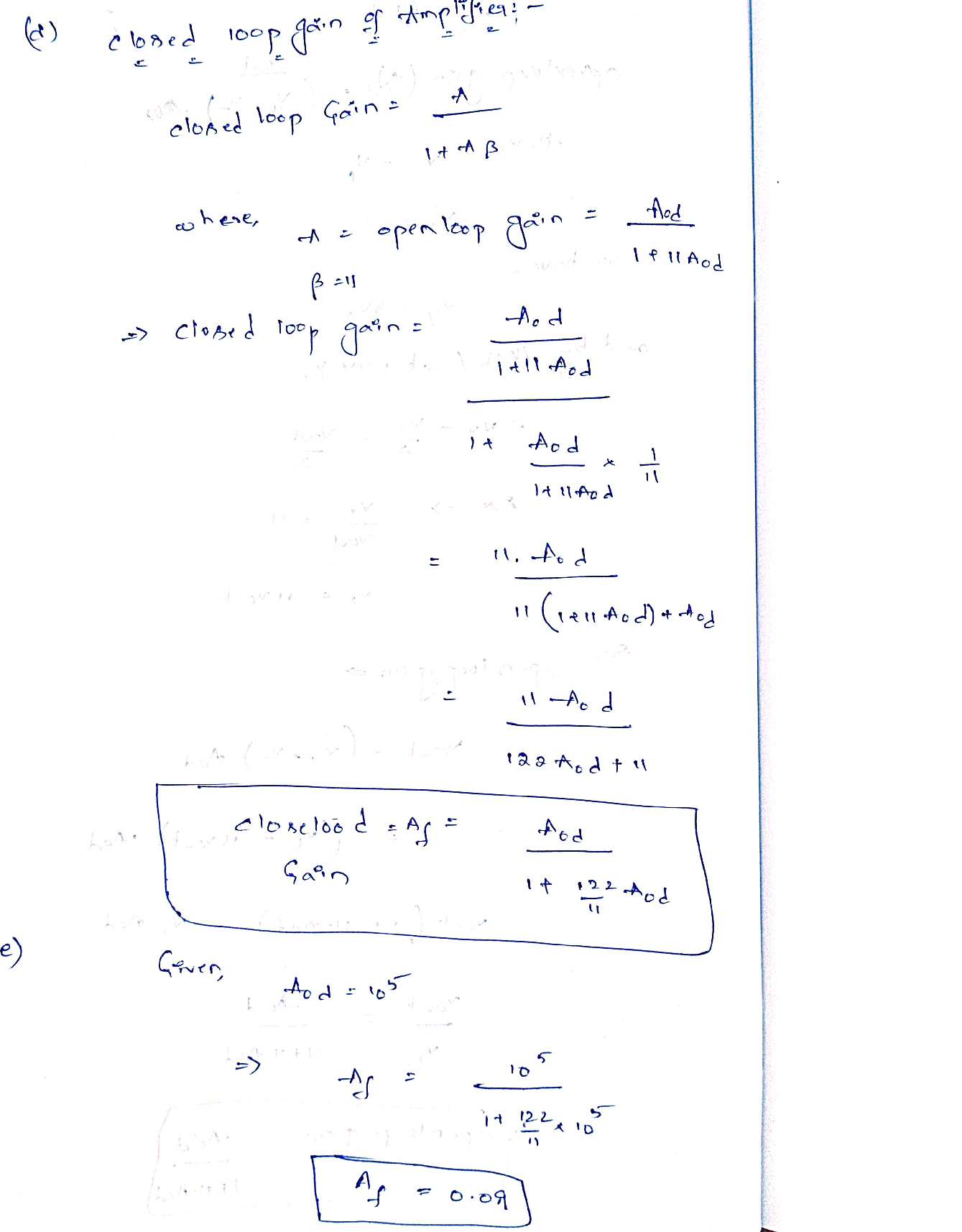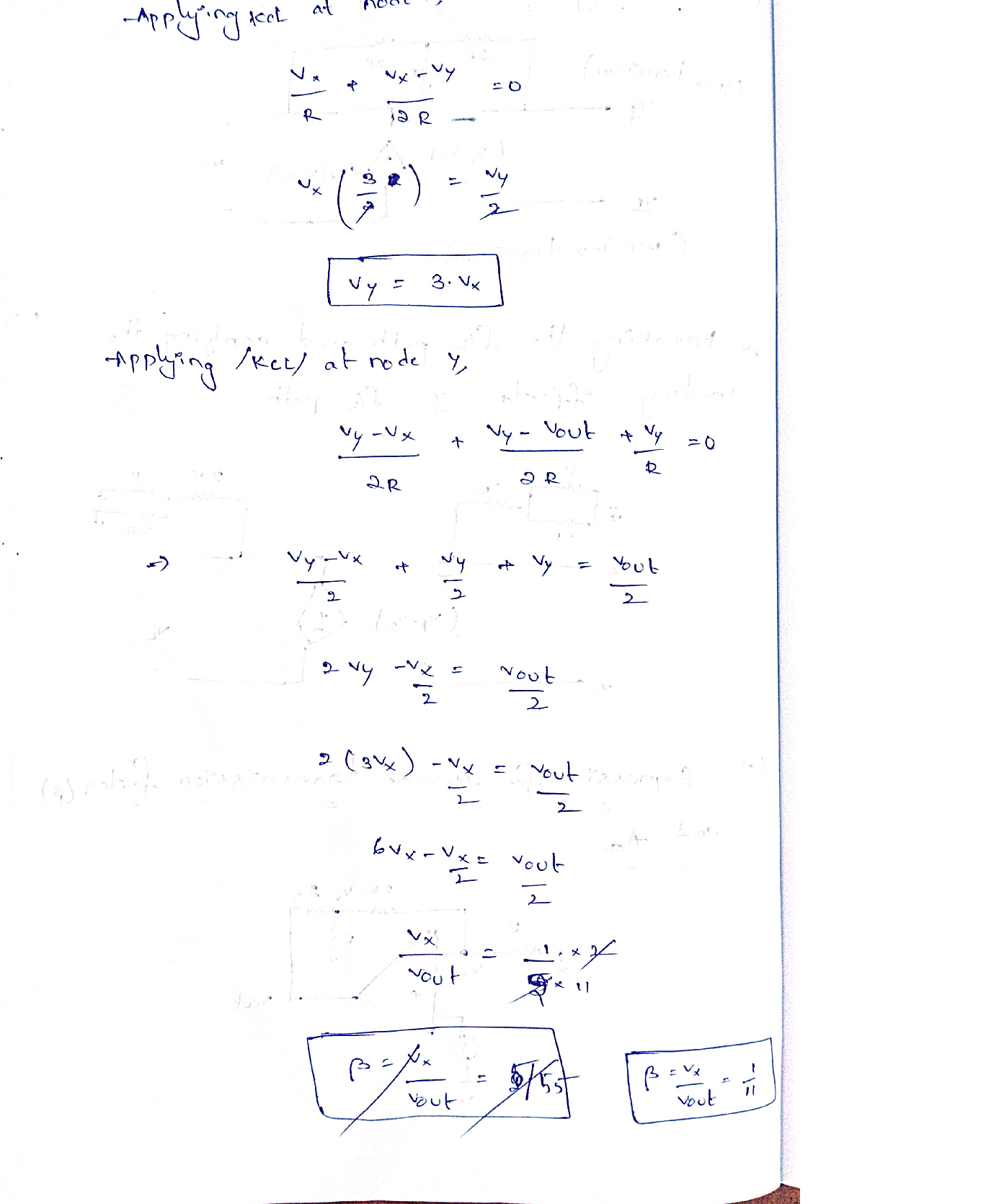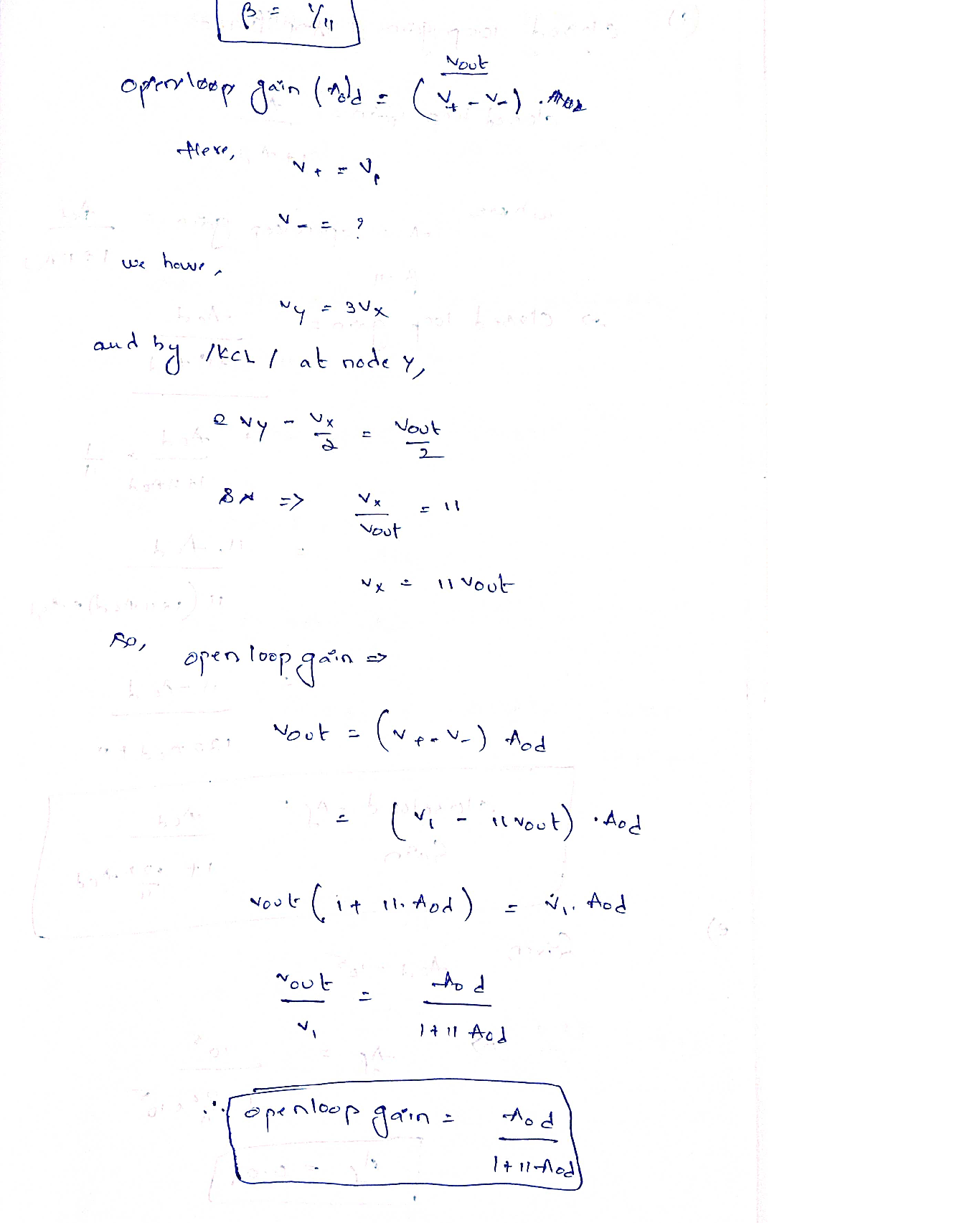### Question 44399Signals And Systems

Problem Statement-A common emitter amplifier as shown has the following design parameters:BIT intrinsic narameters.
\beta_{\mathrm{f}}=80, \mathrm{r}_{\pi}=1600 \Omega, \mathrm{C}_{\pi}=10 \mathrm{pF}, \mathrm{C}_{\mu}=10 \mathrm{pF}
External resistors are-
R_{s}=200 \Omega, R_{1}=7000 \Omega, R_{2}=4500 \Omega, R_{t}=300 \Omega, R_{c}=5 \mathrm{k} \Omega, R_{t}=5 \mathrm{k} \Omega
External capacitors
C_{1}=10 \mu \mathrm{F}, C_{2}=2 \mu \mathrm{F}, C_{E}=100 \mu \mathrm{F}, C_{x}=10 \mathrm{pF}
Use short-circuit zero value single time-constant method to calculate
The high frequency poles
An estimate of the lower 3-dB frequency, fi.
Midband gain vo/vs
Low frequency poles due to the three capacitors C1, C2, and CE
An estimate of the 3-dB frequency, fu

### Question 44070Signals And Systems

\text { For the common-source amplifier in the Figure, } g_{m}=2 \frac{\mathrm{mA}}{V} \text {, and } C_{g s}=20 \mathrm{fF} \text { and } C_{g d}=5 \mathrm{fF} \text {. }
Draw the small signal equivalent circuit at mid-band and find the mid-band gain Am
Draw the small-signal equivalent circuit at low frequency and calculate F,.
Draw the small-signal equivalent circuit at high frequency and calculate FH.

### Question 44059Signals And Systems

a) Sketch the following signals over the period n30 to n-5, showing the exact values foreach value of 'n':
\text { i) } x[n]=n+n^{2}
\text { ii) } x[n]=\cos (n .2 \pi)
\text { iii) } x[n]=n \cdot \cos (n .3 \pi)
In each case state whether you think the signal is random, periodic, or non-random-non-periodic.
b) For the system shown in Figure 2.b below write down the LDE. Re-draw the systemfully labelling all the arrows. State whether the system is IIR or FIR.Then, using a table approach, find the output sequence (y[n]) when the input sequenceX[n] = 8[n]. Evaluate the energy in the sequence y[n] over the first 5 sequence values.%3D
c) Using a table approach, find the sequence h[n] for the system shown in Figure 20below.
Find the TOTAL energy in h[n] and express this energy's a formula and then evaluate it as a number. Is the sequence h[n] an energy or a power signal?

### Question 40942Signals And Systems

5. Using the feedback analysis method, answer the following questions for each circuit:
a.Classify the feedback topology and the type of amplifier.
b. Draw the small-signal equivalent circuit used for calculating the open-loop gain,input resistance, and output resistance, i.e., break the feedback path and include the loading effects of the feedback network.
c. Write expressions for the circuit's reverse transmission factor, B, and open-loop gain, A.
d. Assuming that the circuit has sufficient loop gain, write the approximate expression for the amplifier's closed loop gain, As, and evaluate it.
e. Based on your answer to part d, approximate the feedback amplifier's voltage gain.

### Question 40941Signals And Systems

4. A 3-pole feedback amplifier has a gain and phase response as shown below. Forthe statements given, indicate whether true (T) or false (F) for this amplifier?
\text { At } \omega=\omega_{1} \text { the system is oscillatory }
\text { The system is unconditionally stable }
\text { At } \omega=\omega_{1} \text { the phase margin is } 0^{\circ}
\text { At } \omega=\omega_{p 2} \text { the system is stable }

### Question 40940Signals And Systems

\text { 3. In this circuit, all transistors are matched with } k_{n}=k_{p}=2 \frac{m a}{v^{2}}, V_{t}=0.4 \mathrm{~V} \text { and } V_{A}
a. If VG1 = VG2 = OV, find the dc bias current in each of the four transistors (Q1.2,3.4)as well as the overdrive voltage for each. Ignore the early effect. Also, find the DCvoltage at the sources of Q1 and Q2, as well as, the dc voltages at the gates of Q3and Q4-
b. Find the transconductance gm of each Q1 and Q2 and determine the ro of Q3and Q4.
c. If v1 = +Vid/2 and vg2 = -Vid/2, find the differential gain Ad = Vo/Vid-%3D
d. Find the output resistance of the bias current source Q5 (Rss ) and use it todetermine the common mode gain Acm in the case vG1 = VG2 = Vicm and assume thecurrent mirror has error of 1 % in current transfer ratio. Express the CMRR in dB.%3D
e. If it is required to raise the CMRR by 6 dB, by what factor should the channellength of Q5 be changed?

### Question 40939Signals And Systems

2. Consider a current mirror used in IC construction. Which of the followingstatements are true, and which are false? Indicate your answers by marking a "T"or "F" by each statement.
a. An ideal current source should have an infinite output impedance._
b. When using a FET for a current mirror, wealways want to use it in the trioderegion.
C. Active loads are ideal for an amplifier gain cell as they are a near zero-impedance load.
d. A differential pair amplifier configuration has an ideal gain of zero (dB) for signalsof the same potential at its inputs.
e. In a BJT current mirror, one of the sources of error between the reference andoutput current results from a finite amount of base current that is derived fromone side of the mirror.
f. The current mirror configuration shown is a "current sink",
g. Given a MOSFET with characteristic curves as shown. You are to use this devicefor a current mirror. Operating the device at a Vgs of 10v is a better choice than aVgs of 3v.

### Question 40938Signals And Systems

1. Consider negative feedback applied to a current amplifier. Which of the following statements are true, and which are false? Indicate your answers by marking a "T" or "F" by each statement.
a. The appropriate feedback topology for this case is shunt-series.
b. With feedback applied to the circuit, the input resistance is increased.
c. With feedback applied to the circuit, the current gain and any associated fluctuations are desensitized.
d. With feedback, the circuit has a larger output resistance.
e. Feedback has a negligible effect on the amplifier's bandwidth.

### Question 40103Signals And Systems

Three common types of ADC architectures are the Successive ApproximationRegister (SAR) ADC, the flash ADC, and the sigma delta ADC. A particularapplication requires 10-bit digitisation at a sampling rate of 1 MHz.
A. A flash ADC is B.recommended as it is needed to sample at1 MHz
A typical SAR ADC is recommended as it within specification and has the lowest cost
C.B. A sigma delta ADC is recommended as it is needed to get 10-bit digitisation
D. None of these

### Question 40102Signals And Systems

For this 2 op-amp instrumentation amplifier,R1 = 10 k2ohms and R2 = 250 kohms.The external gain resistor, RG is not connected. What is the gain of the amplifier?

### Submit query